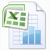# Using the SUMIF Excel Function to add desired vañues that mathc certain criteria.Easily add values of certain cells meeting certain cirteria.
Català – Castellano – Deutsch
Microsoft Excel’s SUMIF function enables you to add the values in a range of cells that satisfy particular requirements. It is an efficient technique for computing the total of particular pieces of information included in a bigger dataset.

The syntax for the SUMIF function is as follows:

=SUMIF(range, criteria, [sum_range])

range denotes the range of cells to which the criteria should be applied.
criteria is the requirement that cells in the range must satisfy in order to be included in the sum.
The range of cells you want to sum is specified by the optional parameter [sum range]. The range argument is utilized as the sum range if this parameter is omitted.

Here is an example of how to total the values in a range of cells based on a particular criterion using the SUMIF function:
Consider a data table where column A is a list of names and column B is a list of values. For all rows with the name…